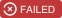# bookshelf-scopes

Giving you Rails like scopes in Bookshelf.js.

## Stats

StarsIssuesVersionUpdatedCreatedSize
bookshelf-scopes
1431.5.15 years ago9 years agoBookshelf-ScopesGiving you Rails like scopes in Bookshelf.js. This was originally a PK4Media project but I moved it over to my account as they do not use it anymore.
If you add in the plugin like so:
``````var bookshelf = require('bookshelf')(knex);

bookshelf.plugin(require('bookshelf-scopes'));``````

You will then be able to add a scopes property on your models that will give you a Knex query builder as the first argument and then followed by any additional arguments. See examples below.
Also just like rails we can set a default. See examples below.

## Examples

### Simple

You can define a model with scopes and an active function like this:
``````var TestModel = bookshelf.Model.extend({
tableName: 'testmodel',
scopes: {
active: function(qb) {
qb.where({status: 'Active'});
},
nameContains: function(qb, name) {
qb.where(knex.raw('name LIKE ?', '%' + name + '%'));
}
}
});``````
You can now run code like this to get all Active:
``````TestModel.active().fetchAll().then(function(allActiveTests) {
...
});``````
You can also get all active where name contains test as well:
``````TestModel.active().nameContains('test').fetchAll().then(function(allActiveTests) {
...
});``````

### Default

You can define a model with scopes and default like this:
``````var TestModel = bookshelf.Model.extend({
tableName: 'testmodel',
scopes: {
default: function(qb) {
qb.where({archived: 0});
}
}
});``````
Now if you call fetchAll or fetch on any of your queries you will only get items that have archive set to 0:
``````TestModel.fetchAll().then(function(allUnArchived) {
...
});``````

If you need to query without the default scope you can call unscoped like so:
``````TestModel.unscoped().fetchAll().then(function(allModels) {
...
});``````

### Combine Methods In Scope

You can define a bunch of scope functions you can also combine them in another scope function.
``````var TestModel = bookshelf.Model.extend({
tableName: 'testmodel',
scopes: {
running: function(qb) {
qb.where({running: 0});
},
byDate: function(qb, date) {
qb.where('created_date', '>=', date);
},
runningByDate: function(qb, date) {
this.running(qb);
this.byDate(qb, date);
}
}
});``````
Now you can use the combined scope method as well to make things more readable.
``````TestModel.runningByDate('2015-01-01').fetchAll().then(function(allUnArchived) {
...
});``````

### Scopes on Relationships

You can also use scopes in the relationships as well. So you could have a model like this:
``````var TestModel = bookshelf.Model.extend({
tableName: 'testmodel',
scopes: {
active: function(qb) {
qb.where('active', '=', true);
}
}
});``````
And then you can have a model that has many active models above as children like so:
``````var MyModel = bookshelf.Model.extend({
tableName: 'mymodel',
active_test_models: function() {
return this.hasMany(TestModel1).active();
}
});``````

### Override Initialize

If in your model you set an initialize you will need to call addScope() to add default scope if you want it
``````var TestModel = bookshelf.Model.extend({
tableName: 'testmodel',
scopes: {
default: function(qb) {
qb.where({status: 'Active'});
}
},
initialize: function() {
this.addScope(); //Now default scope is set, all other scopes work regardless.
this.newValue = 1;
}
});``````

Then calls to fetchAll will include it.
``````TestModel.fetchAll().then(function(allActive) {
...
});``````